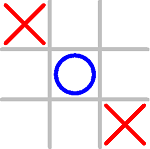## Tic-Tac Toe Game using C Programming

Posted by Tushar Bedekar/* A simple Tic Tac Toe game. */

#include <stdio.h>
#include <stdlib.h>

char matrix;                   /* the tic tac toe matrix */
char check(void);
void init_matrix(void);
void get_player_move(void);
void get_computer_move(void);
void disp_matrix(void);
int main(void)
{
char done;
printf("This is the game of Tic Tac Toe.\n");
printf("You will be playing  against the computer.\n");
done = ' ';
init_matrix();
do{
disp_matrix();
get_player_move();
done = check();                                                               /* see if winner */
if(done!= ' ') break;                                                       /* winner!*/
get_computer_move();
done = check();                                      /* see if winner */
} while(done== ' ');
if(done=='X') printf("You won!\n");
else printf("I won!!!!\n");
disp_matrix();                                          /* show final positions */
return 0;
}

/* Initialize the matrix. */

void init_matrix(void)
{
int i, j;
for(i=0; i<3; i++)
for(j=0; j<3; j++) matrix[i][j] = ' ';
}

/* Get a player's move. */

void get_player_move(void)
{
int x, y;
printf("Enter X,Y coordinates for your move: ");
scanf("%d%*c%d", &x, &y);
x--; y--;
if(matrix[x][y]!= ' '){
printf("Invalid move, try again.\n");
get_player_move();
}
else matrix[x][y] = 'X';
}

/* Get a move from the computer. */

void get_computer_move(void)
{
int i, j;
for(i=0; i<3; i++){
for(j=0; j<3; j++)
if(matrix[i][j]==' ') break;
if(matrix[i][j]==' ') break;
}
if(i*j==9) {
printf("draw\n");
exit(0);
}
else
matrix[i][j] = 'O';
}

/* Display the matrix on the screen. */

void disp_matrix(void)
{
int t;
for(t=0; t<3; t++) {
printf(" %c | %c | %c ",matrix[t],
matrix[t], matrix [t]);
if(t!=2) printf("\n---|---|---\n");
}
printf("\n");
}

/* See if there is a winner. */

char check(void)
{
int i;
for(i=0; i<3; i++)                                                    /* check rows */
if(matrix[i]==matrix[i] &&
matrix[i]==matrix[i]) return matrix[i];
for(i=0; i<3; i++)                                                   /* check columns */
if(matrix[i]==matrix[i] && matrix[i]==matrix[i])
return matrix[i];
/* test diagonals */
if(matrix==matrix &&
matrix==matrix)
return matrix;
if(matrix==matrix &&
matrix==matrix)
return matrix;
return ' ';
}